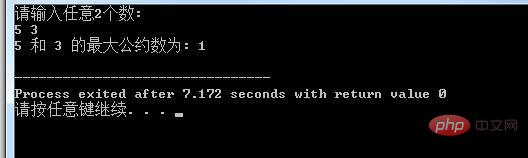# C语言计算两个数的最大公约数和最小公倍数```#include<stdio.h>
int main()
{
int m, n, temp, i;
printf("请输入任意2个数:\n");
scanf("%d%d", &m, &n);
if(m<n)  /*比较大小，使得m中存储大数，n中存储小数*/
{ /*交换m和n的值*/
temp=m;
m=n;
n=temp;
}
for(i=n; i>0; i--)  /*按照从大到小的顺序寻找满足条件的自然数*/
if(m%i==0 && n%i==0)
{/*输出满足条件的自然数并结束循环*/
printf("%d 和 %d 的最大公约数为: %d\n", m, n, i);
break;
}

return 0;
}``````#include<stdio.h>
int main()
{
int m, n, temp, i;
printf("请输入任意2个数:\n");
scanf("%d%d", &m, &n);
if(m<n)  /*比较大小，使得m中存储大数，n中存储小数*/
{ /*交换m和n的值*/
temp=m;
m=n;
n=temp;
}
for(i=m; i>0; i++)  /*从大数开始寻找满足条件的自然数*/
if(i%m==0 && i%n==0)
{/*输出满足条件的自然数并结束循环*/
printf("%d 和 %d 的最小公倍数为: %d\n", m, n, i);
break;
}

return 0;
}```●　最小公倍数=两数的乘积/最大公约（因）数